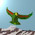## Tuesday, December 13, 2011

### Through The Looking Glass II

Let us say this as simply as possible. Here we use the ordinary quantum Fourier transform, not the twisted version (which sends one neutrino to the photon).

In passing the looking glass, the neutral particles are special. Recall that the set of charged leptons and quarks can be sent through a mirror set in two ways. One can (i) flip charges on all ribbons, by flipping the $B_2$ crossings, or (ii) flip the two crossings in the $B_3$ braid. In case (i) the neutrino sector is unaltered, but the $Z$ color triplets cross the mirror. In case (ii) the $Z$ triplets are unaltered, because they are bosons, but the neutrino sector is swapped.

In both cases, the photon is fixed at the mirror. The fun Fourier transform sends neutrino operators to either photons or $Z$ bosons, and vice versa. Thus (i) and (ii) are just two ways of looking through the glass. So we expect the relation between $Z$ bosons and photons to resemble the relation between neutrinos, mirror neutrinos and photons.

Altogether there are $12$ neutrino states, including generations, and $12$ $Z$ bosons, including color. This defines a $24$ dimensional space with Fourier supersymmetry. The $24$th roots of unity ($\pm \pi /12$) denote this arithmetic dimension. They can only appear as Koide phases for the neutrinos, because $Z$ bosons are diagonal. The left right pairing of braids to create rest mass is the color kinematic duality of Bern et al.

#### 4 comments:

1.And there are no fairies!!

2.I just commented at vixra that there may be reasons to obtain "something" at 126 GeV, without presupposing the Higgs mechanism for mass in general. I mentioned two considerations, the fact that this mass is 1/2 the sum of W+, W-, and Z masses, and the possibility that having a ubiquitous Higgs-like energy density of 246 GeV (the Higgs VEV) may also be enough to obtain a "self-interaction" of this energy density arising solely from virtual processes (see the part about radiative corrections to a Higgs field with no self-coupling at the Planck scale).

So if this holds up, you may want to examine the idea that what is being seen is a virtual process which involves a superposition (or stacking or other combination) of the W and Z braids.

3.... and in which Louise's conservation law is at work, I meant to add.

4.That's a good point, Mitchell. But I will wait until tomorrow and see. If anything is there, which I don't for one second believe, the publicity machine will call it the Higgs.

As you can see in this post, our diagonal tetractys paths are not yet fully explained. But in the bioctonion algebra, they are complex numbers, as opposed to bioctonions. All localized-in-3D states observed so far (and a long time ago) have been in the bioctonions. The complex diagonal suggests something localized-in-1D, if anything. It manifests measurement itself.

Moreover, if we collapse the square faces of the permutohedron we get triangle faces on an octahedron. Thus we can relabel the tetractys just using the known quarks and leptons, without anything extra at all ...

Note: Only a member of this blog may post a comment.